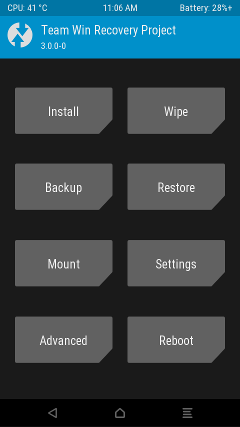## 刷机流程

``````graph LR
B --> C(双清数据)
C --> D(挂载手机存储)
D --> E(导入ROM)
E --> F(安装ROM)``````

``````graph LR
A(设置) --> B(我的设备)
B --> C(全部参数)
C --> D(MIUI版本)
D --点击7下--> E(开发者模式)``````

## 刷入 TWRP

TWRP 本质是一个 Recovery 镜像，可以方便用户对设备数据进行操作，功能界面如下• 系统安装
• 数据擦除
• 数据备份及恢复
• 手机存储挂载

TWRP 设备支持页面中找到自己的手机型号，进入对应的设备页面，会有相关的安装介绍，每个设备的安装方式也许会有差异，但核心流程都是

``````graph LR
A(下载 TWRP 镜像) --> B(写入设备)``````

## 双清数据

``````graph LR
A(右滑进入主页面) --> B(Wipe)
B --> C(Format data)
C --> D(右滑确认操作)
D --> E(点击左上角图标回到主页面)``````